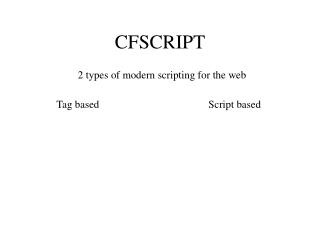# CFSCRIPT - PowerPoint PPT PresentationDownload PresentationCFSCRIPT

Presentation Description
Download Presentation## CFSCRIPT

- - - - - - - - - - - - - - - - - - - - - - - - - - - E N D - - - - - - - - - - - - - - - - - - - - - - - - - - -
##### Presentation Transcript

1. CFSCRIPT 2 types of modern scripting for the web Tag based Script based

2. CFSCRIPT • Block tags • Whatever is inside will be executes (evaluation zone) • No tags allowed • Each expression must be terminated by a semi-colon (;) • Assignment is simply variablename=value String Number Function Operation Expression <CFSCRIPT> Name="Michael"; Age=29; Date=Now(); Sum=11+7; AgeNext=Age+1; </CFSCRIPT>

3. CFSCRIPT • 2 forms of comments • Comments needed as the code will be more obfuscated <CFSCRIPT> // This is a one line comment. Use this to comment on a code snippet /* This is a multi-line comment Use this when writing docs at the top of a CFACRIPT block */ </CFSCRIPT>

4. CFSCRIPT • Execution functions (see handout) DO NOT NEED to be assigned to a variable, especially in CFSCRIPT <CFSCRIPT> // This will clear the session structure StructClear(session); // This will disconnect the data sources cfusion_dbconnections_flush(); // Reset a query cell QuerySetCell(myquery, 'name', 'Michael', 1); </CFSCRIPT>

5. CFSCRIPT • Pay attention to standard Reserve Words • New Reserve Words

6. CFSCRIPT: If <CFSCRIPT> // Standard IF statment IF (score GT 1) result = "positive"; // If statment with multiple parts in the if IF (score GT 1) { result = "positive"; totalscore=score+1; } </CFSCRIPT> Statment }code block - seen as one complete unit

7. CFSCRIPT: IF - Else <CFSCRIPT> // If with an Else IF (score GT 1) result = "positive"; ELSE result = "negative"; // If, Else, Else If IF (score GT 1) result = "positive"; ELSEIF (score EQ 0) result="null"; ELSE result = "negative"; </CFSCRIPT> }Sub IF statment

8. CFSCRIPT: Switch <CFSCRIPT> switch(name) { case "John": { male=true; break; } case "Mary": { male=false; break; } default: { found=false; break; } } //end switch </CFSCRIPT>

9. CFSCRIPT: For Loop • For loop • FOR (init-expr ; test-expr ; final-expr) statement ; • init-expr and final-expr can be • single assignment expression, for example, x=5 or loop=loop+1 • any ColdFusion expression, for example, SetVariable("a",a+1) • empty • The test-expr can be • any ColdFusion expression, for example, A LT 5, loop LE x, or Y EQ "not found" AND loop LT • empty

10. CFSCRIPT: For Loop <CFSCRIPT> // Loop from 1 to 10 for(Loop1=1; Loop1 LT 10; Loop1 = Loop1 + 1) score=score+loop1; // Loop from 1 to 10 for(Loop1=1; ; Loop1 = Loop1 + 1) { score=score+loop1; IF (Loop1 EQ 10) break; } </CFSCRIPT>

11. CFSCRIPT: While Loop <CFSCRIPT> // While expression is true a = ArrayNew(1); while (loop1 LT 10) { a[loop1] = loop1 + 5; loop1 = loop1 + 1; } // While expression is true a = ArrayNew(1); while (loop1 LT 10) { IF (loop1 mod 2 EQ 0) continue; loop1 = loop1 + 1; } </CFSCRIPT>

12. CFSCRIPT: Do-While Loop <CFSCRIPT> // Multiline do-while loop // Tests AFTER code is run a = ArrayNew(1); do { a[loop1] = loop1 + 5; loop1 = loop1 + 1; } while (loop1 LT 10); </CFSCRIPT>

13. CFSCRIPT: For-In Loop Special loop for dealing with collections (I.e. structures) for (variable in collection) statement ; <CFSCRIPT> for (x in Session) WriteOutPut(Session[x]&’<br>’); </CFSCRIPT>